# 1   Introduction

Temporal drift in X-ray source positions can occur due to changes in the periscope alignment which induce drift in fid light positions as observed by the Aspect camera. Further analysis of the periscope alignment drift reveals that approximately 300 observations may be affected with drifts of more than 0.1 arcseconds.

A pre-launch calibration of the periscope gradients to periscope alignment does exist, but we performed a recalibration using on-orbit data that reveals that the pre-launch coefficients may be off by up to a factor of 10. This new calibration is used in this tool to correct fid light positions based on telemetered periscope gradients. A detailed algorithm specification is available as python prototype code.

# 2   Calibration Model

We constructed two models (one in y-angle and one in z-angle). Each model is a simple linear combination of the two periscope thermal gradients:

OOBAGRD3 (~axial) OOBAGRD6 (~diametrical)

Y-angle model:

dy = cya * OOBAGRD3 + cyd * OOBAGRD6

Z-angle model:

dz = cza * OOBAGRD3 + czd * OOBAGRD6

# 3   Calibration Method

We found a set of 175 long (>40ks) science observations, each with a bright (>2000 counts), on-axis (small detsize) source. From this set, we selected the 16 observations with the largest changes in the periscope gradients. These 16 were used as a fit set, and we determined new values for cya, cyd, cza, and cyd by performing a simultaneous fit of the model to all of the events in each of these observations. Specifically, for each observation we:

• Extracted X-ray events in a small circular region centered on a point source.
• Transformed the position of each event from RA, Dec to Y-ang, Z-ang
• Created a Sherpa User Model defined as mentioned above, using values of OOBAGRD3 and OOBAGRD3 interpolated at each X-ray event time
• Fit all of the mean-subtracted X-ray events in yag and zag to the mean-subtracted values of the model to determine new simple coefficients cya, cyd, cza, and czd.

For the remaining observations, we calculated the model residuals against the event data (using the mean of 10 ks bins in each observation) to evaluate the fit.

# 4   Fit Result

The by-obsid plots of the model and the position data confirm that the fit is reasonable.

## Y-Axis Example: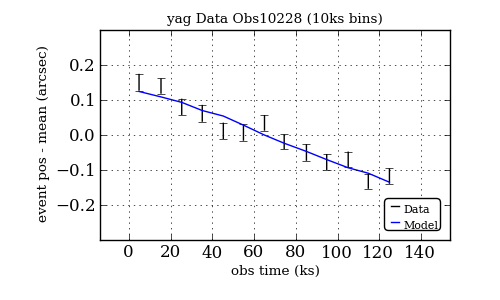## Z-Axis Example: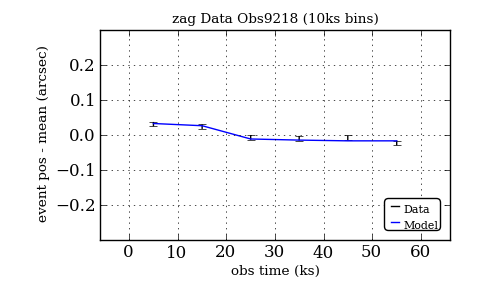Additionally, the residual histograms of the fit demonstrate that there is a significant useful correction in Y-angle and a small improvement in Z-angle.

Y-Angle Data and Corrected Data Z-Angle Data and Corrected Data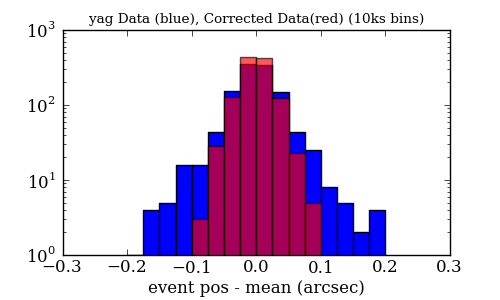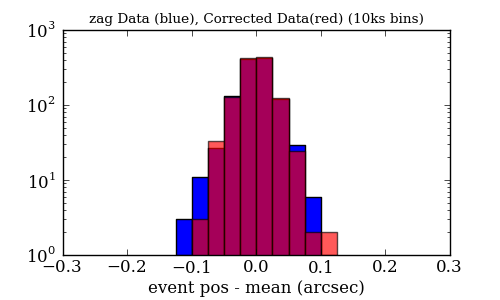# 5   Pipeline Correction Implementation

Following the model of the ACIS DH fid correction process, we again propose to correct the observed (smoothed) fid light centroids in place. The correction code is, of course, very close to the fit code. We:

• Determine the mean fid position in Y-angle and Z-angle
• Determine the mean values of the periscope gradients
• Calculate smoothed values of the gradients
• At each centroid time, determine the smoothed - mean values of the gradients
• At each centroid time, subtract the value of the smoothed-mean gradients scaled by the appropriate calibration coefficient

See the python prototype code for the prototype implementation of this algorithm as described.

# 6   Prototype performance

End-to-end testing of the pipeline correction implementation reveals that the correction is applied appropriately and that the corrected binned event positions are centered on the mean position.

Uncorrected Corrected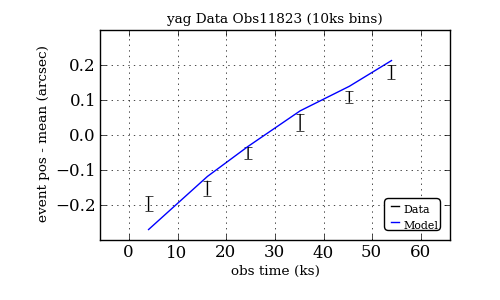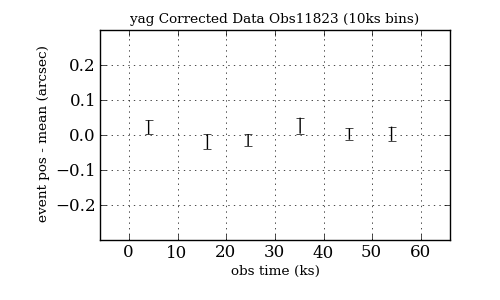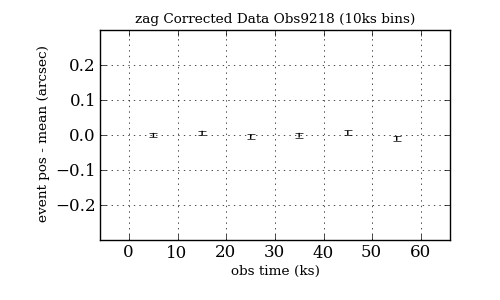# 7   CALDB update

The constants defined in the prototype code could logically be put in a new binary table HDU in the CALDB PCAD CALALIGN product with an extension name PERIFIDCORR.